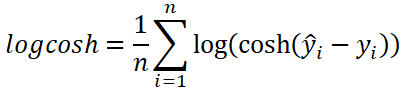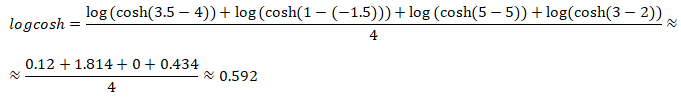# Calculate Log-Cosh Loss using TensorFlow 2

Log-cosh loss is a loss function that is used to solve regression problems. Log-cosh is calculated as the average logarithm of the hyperbolic cosine of the differences between the predicted and actual values.

The formula to calculate the log-cosh:• `n` - the number of data points.
• `y` - the actual value of the data point. Also known as true value.
• `ŷ` - the predicted value of the data point. This value is returned by model.

Let's say we have the following sets of numbers:

Here is example how log-cosh can be calculated using these numbers:TensorFlow 2 allows to calculate the log-cosh. It can be done by using `LogCosh` class.

``````from tensorflow import keras

yActual = [4, -1.5, 5, 2]
yPredicted = [3.5, 1, 5, 3]

logcoshObject = keras.losses.LogCosh()
logcoshTensor = logcoshObject(yActual, yPredicted)
logcosh = logcoshTensor.numpy()

print(logcosh)``````

Log-cosh also can be calculated by using `log_cosh` function.

``````logcoshTensor = keras.losses.log_cosh(yActual, yPredicted)
logcosh = logcoshTensor.numpy()``````